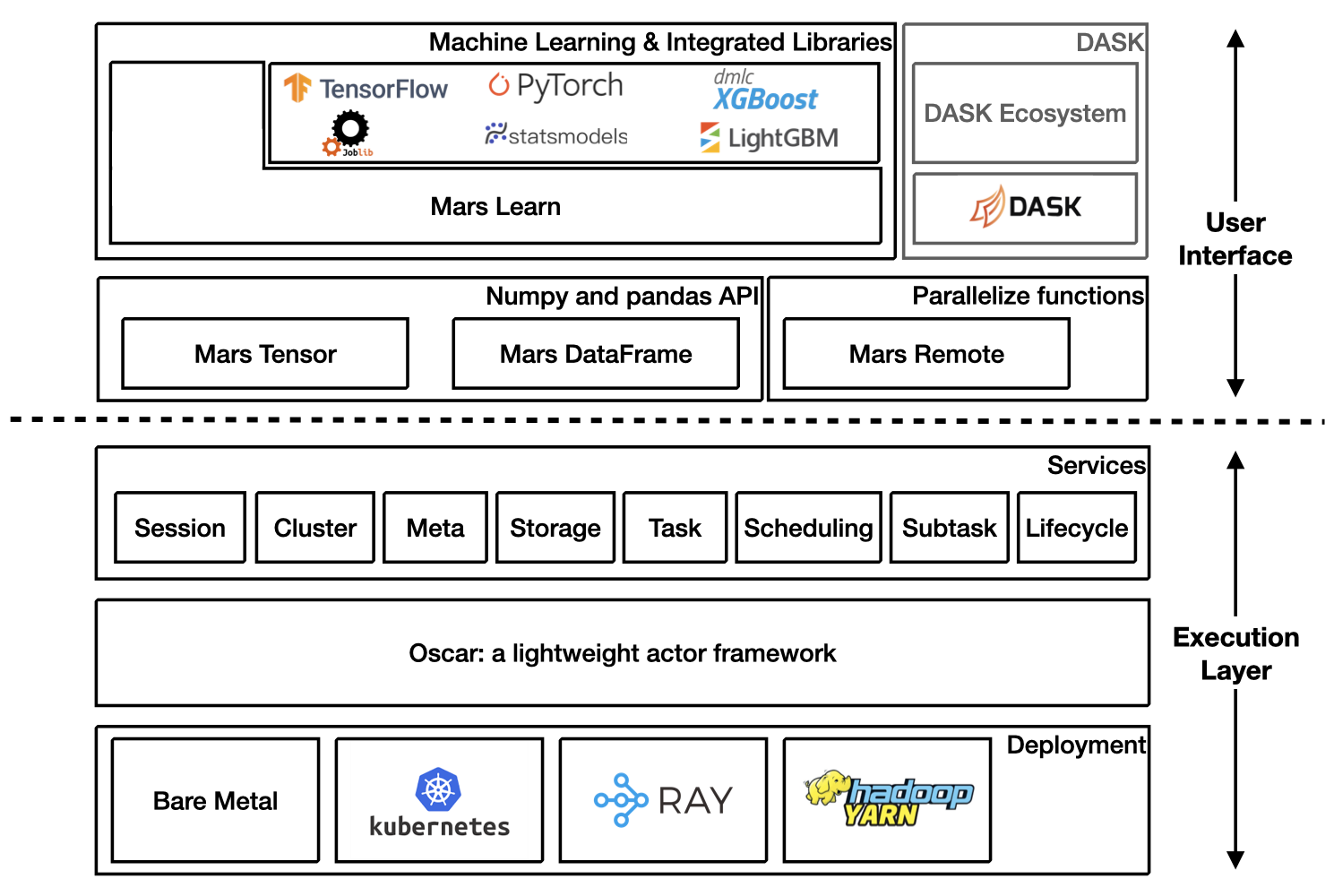Mars is a tensor-based unified framework for large-scale data computation which scales numpy, pandas, scikit-learn and many other libraries.

## Installation

Mars is easy to install by

```pip install pymars
```

### Installation for Developers

When you want to contribute code to Mars, you can follow the instructions below to install Mars for development:

```git clone https://github.com/mars-project/mars.git
cd mars
pip install -e ".[dev]"
```

More details about installing Mars can be found at installation section in Mars document.

## Architecture Overview## Getting Started

Starting a new runtime locally via:

```>>> import mars
>>> mars.new_session()
```

Or connecting to a Mars cluster which is already initialized.

```>>> import mars
>>> mars.new_session('http://<web_ip>:<ui_port>')
```

## Mars Tensor

Mars tensor provides a familiar interface like Numpy.

 Numpy Mars tensor ```import numpy as np N = 200_000_000 a = np.random.uniform(-1, 1, size=(N, 2)) print((np.linalg.norm(a, axis=1) < 1) .sum() * 4 / N) ``` ```import mars.tensor as mt N = 200_000_000 a = mt.random.uniform(-1, 1, size=(N, 2)) print(((mt.linalg.norm(a, axis=1) < 1) .sum() * 4 / N).execute()) ``` ```3.14174502 CPU times: user 11.6 s, sys: 8.22 s, total: 19.9 s Wall time: 22.5 s ``` ```3.14161908 CPU times: user 966 ms, sys: 544 ms, total: 1.51 s Wall time: 3.77 s ```

Mars can leverage multiple cores, even on a laptop, and could be even faster for a distributed setting.

## Mars DataFrame

Mars DataFrame provides a familiar interface like pandas.

 Pandas Mars DataFrame ```import numpy as np import pandas as pd df = pd.DataFrame( np.random.rand(100000000, 4), columns=list('abcd')) print(df.sum()) ``` ```import mars.tensor as mt import mars.dataframe as md df = md.DataFrame( mt.random.rand(100000000, 4), columns=list('abcd')) print(df.sum().execute()) ``` ```CPU times: user 10.9 s, sys: 2.69 s, total: 13.6 s Wall time: 11 s ``` ```CPU times: user 1.21 s, sys: 212 ms, total: 1.42 s Wall time: 2.75 s ```

## Mars Learn

Mars learn provides a familiar interface like scikit-learn.

 Scikit-learn Mars learn ```from sklearn.datasets import make_blobs from sklearn.decomposition import PCA X, y = make_blobs( n_samples=100000000, n_features=3, centers=[[3, 3, 3], [0, 0, 0], [1, 1, 1], [2, 2, 2]], cluster_std=[0.2, 0.1, 0.2, 0.2], random_state=9) pca = PCA(n_components=3) pca.fit(X) print(pca.explained_variance_ratio_) print(pca.explained_variance_) ``` ```from mars.learn.datasets import make_blobs from mars.learn.decomposition import PCA X, y = make_blobs( n_samples=100000000, n_features=3, centers=[[3, 3, 3], [0, 0, 0], [1, 1, 1], [2, 2, 2]], cluster_std=[0.2, 0.1, 0.2, 0.2], random_state=9) pca = PCA(n_components=3) pca.fit(X) print(pca.explained_variance_ratio_) print(pca.explained_variance_) ```

Mars learn also integrates with many libraries:

## Mars remote

Mars remote allows users to execute functions in parallel.

 Vanilla function calls Mars remote ```import numpy as np def calc_chunk(n, i): rs = np.random.RandomState(i) a = rs.uniform(-1, 1, size=(n, 2)) d = np.linalg.norm(a, axis=1) return (d < 1).sum() def calc_pi(fs, N): return sum(fs) * 4 / N N = 200_000_000 n = 10_000_000 fs = [calc_chunk(n, i) for i in range(N // n)] pi = calc_pi(fs, N) print(pi) ``` ```import numpy as np import mars.remote as mr def calc_chunk(n, i): rs = np.random.RandomState(i) a = rs.uniform(-1, 1, size=(n, 2)) d = np.linalg.norm(a, axis=1) return (d < 1).sum() def calc_pi(fs, N): return sum(fs) * 4 / N N = 200_000_000 n = 10_000_000 fs = [mr.spawn(calc_chunk, args=(n, i)) for i in range(N // n)] pi = mr.spawn(calc_pi, args=(fs, N)) print(pi.execute().fetch()) ``` ```3.1416312 CPU times: user 32.2 s, sys: 4.86 s, total: 37.1 s Wall time: 12.4 s ``` ```3.1416312 CPU times: user 616 ms, sys: 307 ms, total: 923 ms Wall time: 3.99 s ```

### Eager Mode

Mars supports eager mode which makes it friendly for developing and easy to debug.

Users can enable the eager mode by options, set options at the beginning of the program or console session.

```>>> from mars.config import options
>>> options.eager_mode = True
```

Or use a context.

```>>> from mars.config import option_context
>>> with option_context() as options:
>>>     options.eager_mode = True
>>>     # the eager mode is on only for the with statement
>>>     ...
```

If eager mode is on, tensor, DataFrame etc will be executed immediately by default session once it is created.

```>>> import mars.tensor as mt
>>> import mars.dataframe as md
>>> from mars.config import options
>>> options.eager_mode = True
>>> t = mt.arange(6).reshape((2, 3))
>>> t
array([[0, 1, 2],
[3, 4, 5]])
>>> df = md.DataFrame(t)
>>> df.sum()
0    3
1    5
2    7
dtype: int64
```

## Mars on Ray

Mars also has deep integration with Ray and can run on Ray efficiently and interact with the large ecosystem of machine learning and distributed systems built on top of the core Ray.

Starting a new Mars on Ray runtime locally via:

```import mars
mars.new_session(backend='ray')
# Perform computation
```

Interact with Ray Dataset:

```import mars.tensor as mt
import mars.dataframe as md
df = md.DataFrame(
mt.random.rand(1000_0000, 4),
columns=list('abcd'))
# Convert mars dataframe to ray dataset
ds = md.to_ray_dataset(df)
print(ds.schema(), ds.count())
ds.filter(lambda row: row["a"] > 0.5).show(5)
# Convert ray dataset to mars dataframe
```

## Easy to scale in and scale out

Mars can scale in to a single machine, and scale out to a cluster with thousands of machines. It's fairly simple to migrate from a single machine to a cluster to process more data or gain a better performance.

### Bare Metal Deployment

Mars is easy to scale out to a cluster by starting different components of mars distributed runtime on different machines in the cluster.

A node can be selected as supervisor which integrated a web service, leaving other nodes as workers. The supervisor can be started with the following command:

```mars-supervisor -h <host_name> -p <supervisor_port> -w <web_port>
```

Workers can be started with the following command:

```mars-worker -h <host_name> -p <worker_port> -s <supervisor_endpoint>
```

After all mars processes are started, users can run

```>>> sess = new_session('http://<web_ip>:<ui_port>')
>>> # perform computation
```

## Getting involved

Get A Weekly Email With Trending Projects For These Topics
No Spam. Unsubscribe easily at any time.
Python (888,324
Machine Learning (40,813
Tensorflow (22,883
Pytorch (22,598
Pandas (7,185
Numpy (6,272
Ray (3,744
Dataframe (2,807
Scikit Learn (2,539
Tensor (2,385
Xgboost (1,737
Lightgbm (638